CBSE Class 12 Sample Paper for 2018 Boards

Class 12
Solutions of Sample Papers and Past Year Papers - for Class 12 Boards

### measured parallel to the plane: x – y + 2z – 3 = 0.

This is a question of CBSE Sample Paper - Class 12 - 2017/18.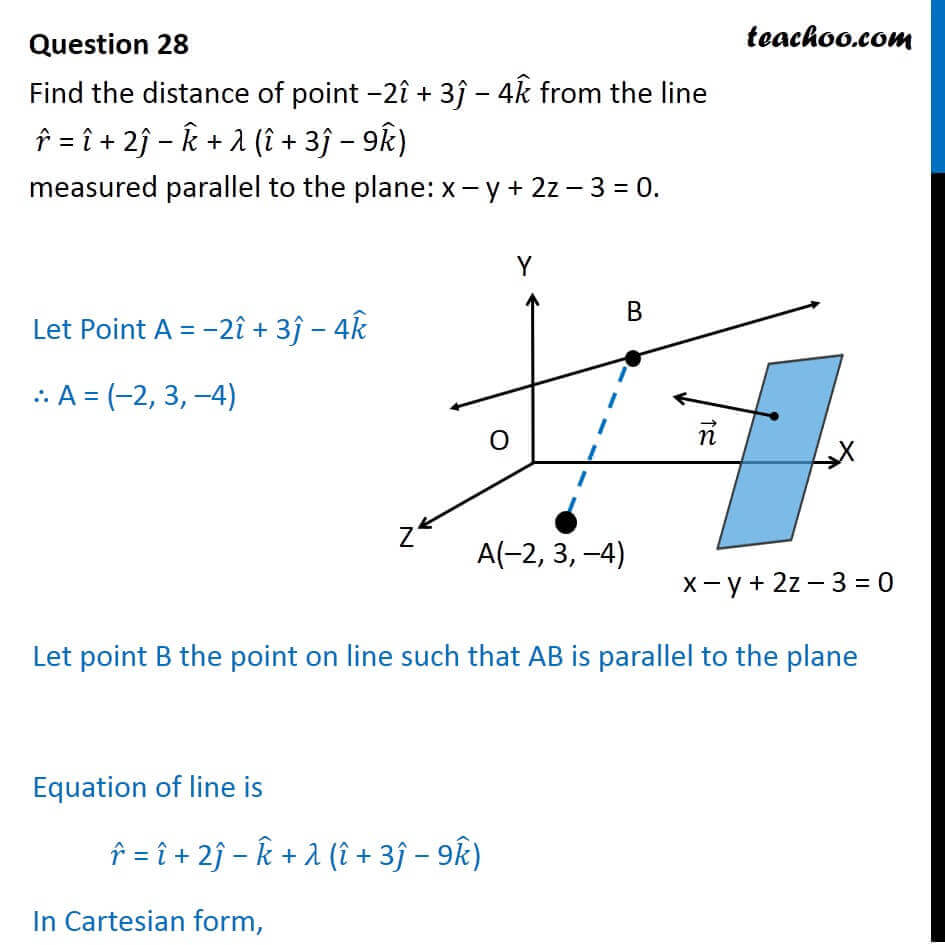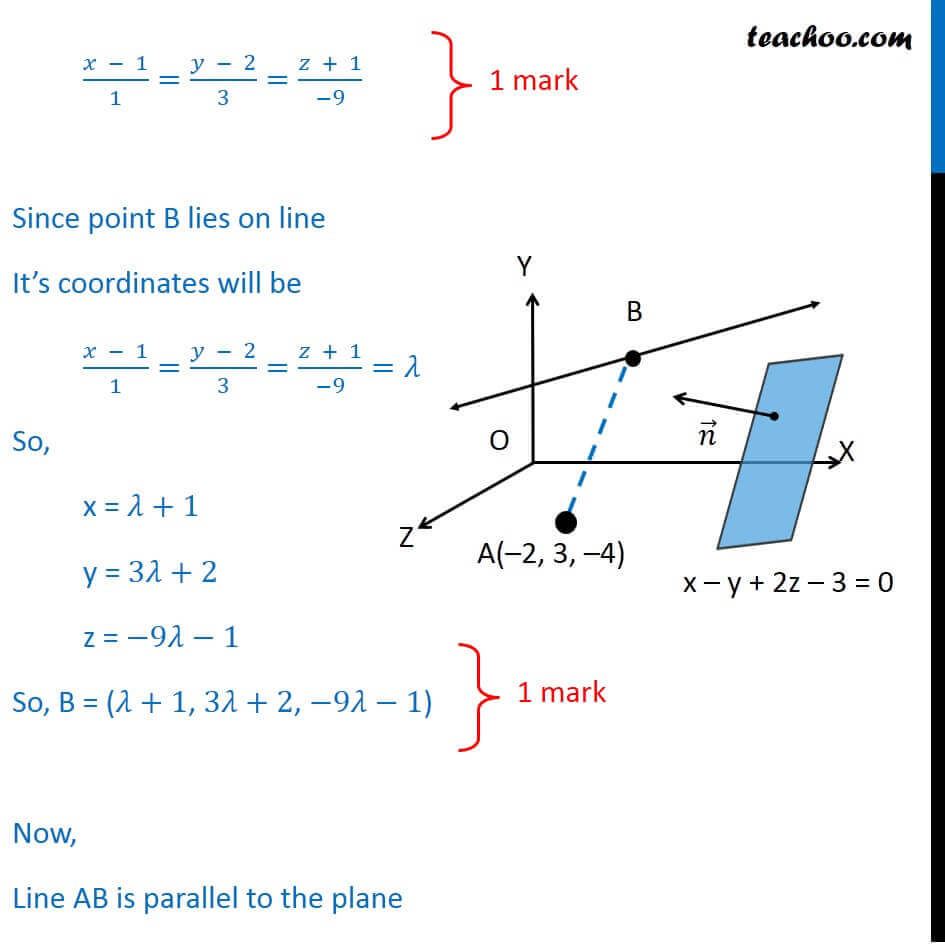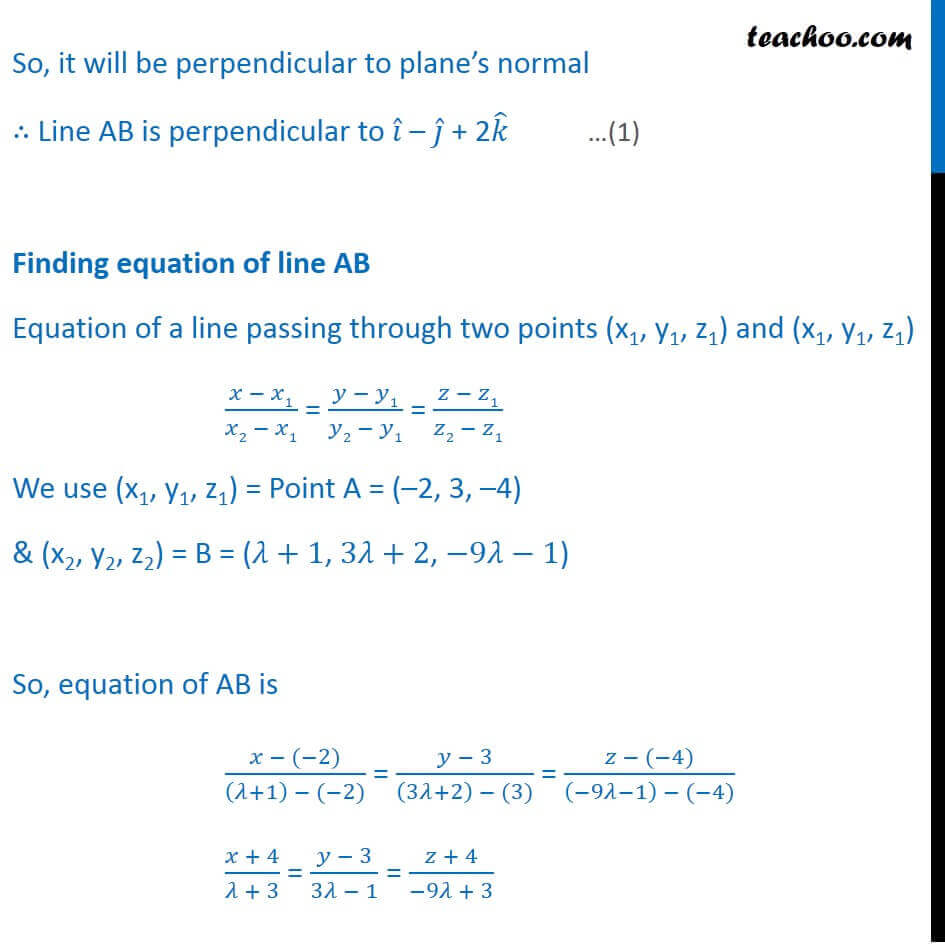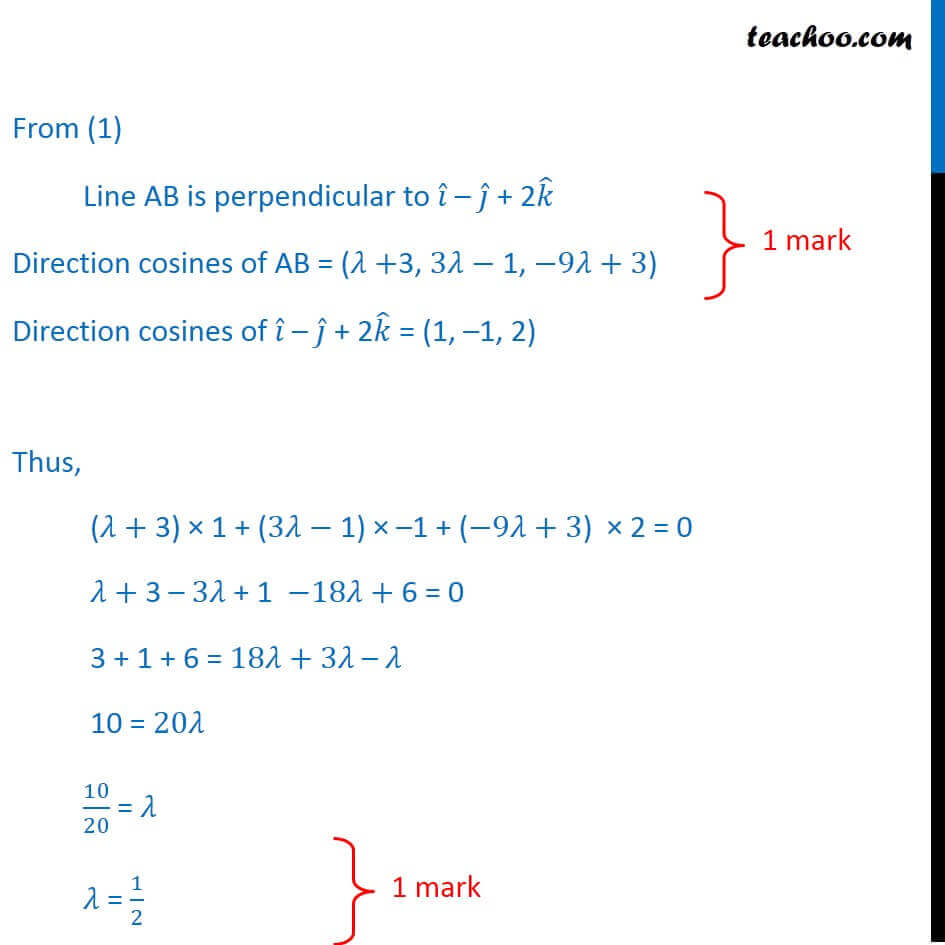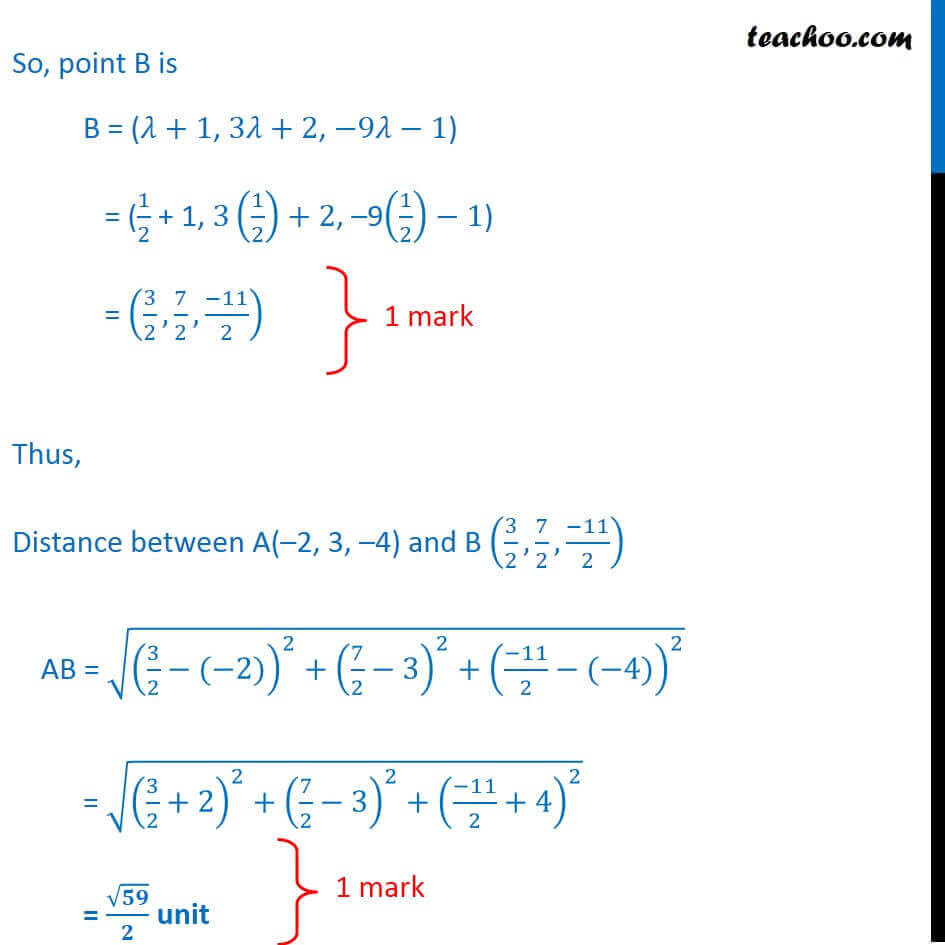Learn in your speed, with individual attention - Teachoo Maths 1-on-1 Class

### Transcript

Question 28 Find the distance of point −2𝑖 ̂ + 3𝑗 ̂ − 4𝑘 ̂ from the line 𝑟 ̂ = 𝑖 ̂ + 2𝑗 ̂ − 𝑘 ̂ + 𝜆 (𝑖 ̂ + 3𝑗 ̂ − 9𝑘 ̂) measured parallel to the plane: x – y + 2z – 3 = 0. Let Point A = −2𝑖 ̂ + 3𝑗 ̂ − 4𝑘 ̂ ∴ A = (–2, 3, –4) Let point B the point on line such that AB is parallel to the plane Equation of line is 𝑟 ̂ = 𝑖 ̂ + 2𝑗 ̂ − 𝑘 ̂ + 𝜆 (𝑖 ̂ + 3𝑗 ̂ − 9𝑘 ̂) In Cartesian form, (𝑥 − 1)/1=(𝑦 − 2)/3=(𝑧 + 1)/(−9) Since point B lies on line It’s coordinates will be (𝑥 − 1)/1=(𝑦 − 2)/3=(𝑧 + 1)/(−9)=𝜆 So, x = 𝜆+1 y = 3𝜆+2 z = −9𝜆−1 So, B = (𝜆+1, 3𝜆+2, −9𝜆−1) Now, Line AB is parallel to the plane So, it will be perpendicular to plane’s normal ∴ Line AB is perpendicular to 𝑖 ̂ – 𝑗 ̂ + 2𝑘 ̂ Finding equation of line AB Equation of a line passing through two points (x1, y1, z1) and (x1, y1, z1) (𝑥 − 𝑥1)/(𝑥2 − 𝑥1) = (𝑦 − 𝑦1)/(𝑦2 − 𝑦1) = (𝑧 − 𝑧1)/(𝑧2 − 𝑧1) We use (x1, y1, z1) = Point A = (–2, 3, –4) & (x2, y2, z2) = B = (𝜆+1, 3𝜆+2, −9𝜆−1) So, equation of AB is (𝑥 − (−2))/((𝜆+1) − (−2)) = (𝑦 − 3)/((3𝜆+2) − (3)) = (𝑧 − (−4))/((−9𝜆−1) − (−4)) (𝑥 + 4)/(𝜆 + 3) = (𝑦 − 3)/(3𝜆 − 1) = (𝑧 + 4)/(−9𝜆 + 3) From (1) Line AB is perpendicular to 𝑖 ̂ – 𝑗 ̂ + 2𝑘 ̂ Direction cosines of AB = (𝜆+3, 3𝜆− 1, −9𝜆+3) Direction cosines of 𝑖 ̂ – 𝑗 ̂ + 2𝑘 ̂ = (1, –1, 2) Thus, (𝜆+ 3) × 1 + (3𝜆− 1) × –1 + (−9𝜆+3) × 2 = 0 𝜆+ 3 – 3𝜆 + 1 −18𝜆+ 6 = 0 3 + 1 + 6 = 18𝜆+ 3𝜆 – 𝜆 10 = 20𝜆 10/20 = 𝜆 𝜆 = 1/2 So, point B is B = (𝜆+1, 3𝜆+2, −9𝜆−1) = (1/2 + 1, 3(1/2)+2, –9(1/2)−1) = (3/2, 7/2,(−11)/2) Thus, Distance between A(–2, 3, –4) and B (3/2, 7/2,(−11)/2) AB = √((3/2−(−2))^2+(7/2−3)^2+((−11)/2−(−4))^2 ) = √((3/2+2)^2+(7/2−3)^2+((−11)/2+4)^2 ) = √𝟓𝟗/𝟐 unit